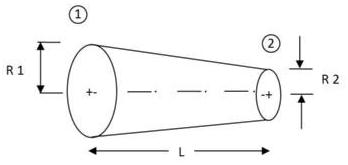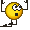# Fluid flow acceleration in a channel

MODERATOR NOTE: THIS HOMEWORK WAS SUBMITTED TO A NON-HOMEWORK FORUM SO THERE IS NO TEMPLATE

Hi everyone,

I'm stuck on a fluid mechanics problem and maybe you could help me.

As shown in figure, there is a two-dimensional converging channel, in which cross-section area varies linearly with x. If Q is the discharge, determine the flow acceleration along the x-axis (A1, A2 and L are known).I got for area: A(x)=(A2-A1/L).x+A1

I'm not sure what to do about Q, should Q=A.v be used? Any hint about how to get the velocity field?

Thanks in advance!

Last edited by a moderator:

## Answers and Replies

boneh3ad
Science Advisor
Gold Member
You don't have any other indication about what they want you to use for discharge? That's an ambiguous term.

Chestermiller
Mentor
At steady state, the acceleration is vdv/dx. Are you familiar with this term in the momentum balance equations?

Chet

At steady state, the acceleration is vdv/dx. Are you familiar with this term in the momentum balance equations?

Chet

Do you mean Navier-Stokes eq.? I still don't know how to get the velocity field...You don't have any other indication about what they want you to use for discharge? That's an ambiguous term.

No, that's all the information. I think discharge here means volume per time. That's why I tried to use Q=A.v

Chestermiller
Mentor
Do you mean Navier-Stokes eq.? I still don't know how to get the velocity field...What about getting v(x) from the two equations in your original post:
A(x)=(A2-A1/L).x+A1
and
Q=A.v

Chet

What about getting v(x) from the two equations in your original post:
A(x)=(A2-A1/L).x+A1
and
Q=A.v

Chet

I thought the same, but isn't v the mean velocity? If I can use v as the v-field, then the acceleration is quite simple via material derivative.

Chestermiller
Mentor
I thought the same, but isn't v the mean velocity? If I can use v as the v-field, then the acceleration is quite simple via material derivative.
It's pretty safe to say that this is what they expected you to do. They just forgot to mention that the channel is converging very gradually and that locally you can assume a flat velocity profile.

Chet

It's pretty safe to say that this is what they expected you to do. They just forgot to mention that the channel is converging very gradually and that locally you can assume a flat velocity profile.

Chet

Wow, so obvious. Thanks Chet.